Students can Download Tamil Nadu 12th Computer Science Model Question Paper 2 English Medium Pdf, Tamil Nadu 12th Computer Science Model Question Papers helps you to revise the complete Tamilnadu State Board New Syllabus and score more marks in your examinations.

## TN State Board 12th Computer Science Model Question Paper 2 English Medium

General Instructions:

1. The question paper comprises of four parts.
2. You are to attempt all the parts. An internal choice of questions is provided wherever applicable.
3. All questions of Part I, II, III and IV are to be attempted separately.
4. Question numbers 1 to 15 in Part I are Multiple Choice Questions of one mark each.
These are to be answered by choosing the most suitable answer from the given four alternatives and writing the option code and the corresponding answer
5. Question numbers 16 to 24 in Part II are two-mark questions. These are to be answered in about one or two sentences.
6. Question numbers 25 to 33 in Part III are three-mark questions. These are to be answered in about three to five short sentences.
7. Question numbers 34 to 38 in Part IV are five-mark questions. These are to be answered in detail Draw diagrams wherever necessary.

Time: 3 Hours
Maximum Marks: 70

PART – I

Choose the correct answer. Answer all the questions: [15 x 1 = 15]

Question 1.
The members that are accessible from within the class and are also available to its subclasses is called……….
(a) public
(b) protected
(c) secured
(d) private
(b) protected

Question 2.
Built in scopes are called as scope.
(a) private
(b) public
(c) protected
(d) module
(d) module

Question 3.
The complexity of Bubble sort is…………
(a) θ (n2)
(b) θ (n(logn)2)
(c) θ (n)
(d) θ (2n)
(a) θ (n2)Question 4.
How many integer data types are there?
(a) 2
(b) 3
(c) 4
(d) 5
(b) 3

Question 5.
Branching statements are otherwise called as………..
(a) alternative
(b) Iterative
(c) loop
(d) sequential
(a) alternative

Question 6.
……….. functions are anonymous un-named functions.
(a) User defined
(b) Built-in
(c) Lambda
(d) Recursive
(c) Lambda

Question 7.
The code block always comes after……..
(a) ;
(b) +
(c) =
(d) :
(d) :

Question 8.
Which one of the following is the membership operator?
(a) is
(b) at
(c) to
(d) in
(d) in

Question 9.
Which operator is used to join two tuples?
(a) –
(b) _
(c) +
(d) +:
(c) +

Question 10.
A table is otherwise called as…………
(a) tuple
(b) relation
(c) attribute
(d) degree
(b) relation

Question 11.
Who developed ER model?
(a) Chen
(b) E F Codd
(c) Chend
(d) Chand
(a) Chen

Question 12.
The latest SQL was released in………
(a) 1987
(b) 1992
(c) 2008
(d) 2012
(c) 2008

Question 13.
How many ways are there to read the CSV files?
(a) 2
(b) 1
(c) 3
(d) 4
(a) 2

Question 14.
CSV files cannot be opened with………
(b) MS Excel
(c) Open office
(d) html
(d) htmlQuestion 15.
getopt mode is given by………..
(a) ;
(b) =
(c) #
(d) :
(d) :

PART – II

Answer any six questions. Question No. 21 is compulsory. [6 x 2 = 12]

Question 16.
List the characteristics of an algorithm.
Input, Output, Finiteness, Definiteness, Effectiveness, Correctness, Simplicity, Unambiguous, Feasibility, Portable and Independent.

Question 17.
What are the escape sequences for Backslash, Newline, Tab, Single quotes.

1. Backslash – \\
2. New line – \n
3. Tab – \t
4. Single quotes – \’

Question 18.
Define composition.
The value returned by a function may be used as an argument for another function in a nested manner. This is called composition.

Question 19.
Name the different types of functions.

1. User-defined Functions
2. Built-in Functions
3. Lambda Functions
4. Recursion Functions

Question 20.
Write note on center function, center (width, fillchar)
Returns a string with the original string centered to a total of width columns and filled with fillchar in columns that do not have characters.

Question 21.
Write a program to create a list of numbers in the range 1 to 20. Then delete all the numbers from the list that are divisible by 3.
num = []
for x in range(1 21):
num.append(x)
print(“The list of numbers from 1 to 20 =”, num)
for index, i in enumerate(num):
if (i % 3 = 0)
del num[index]
print (“The list after deleting numbers”, num)
Output:
The list of numbers from 1 to 20 = [1, 2, 3, 4… 20]
The list after deleting numbers [l, 2, 4, 5, 7, 8, 10, 11, 13, 14, 16, 17, 19, 20]

Question 22.
Write note on dictionary comprehensions.
In Python, comprehension is another way of creating dictionary. The following is the syntax of creating such dictionary.
Syntax
Diet = {expression for variable in sequence [if condition]}

Question 23.
What is data consistency?
On live data, it is being continuously updated and added, maintaining the consistency of data can become a challenge. But DBMS handles it by itself. Data Consistency means that data values are the same at all instances of a database.Question 24.
Define database.
Database is a repository collection of related data organized in a way that data can be easily accessed, managed and updated. Database can be a software or hardware based, with one sole purpose of storing data.

PART – III

Answer any six questions. Question No. 29 is compulsory. [6 x 3 = 18]

Question 25.
Define constructors and selectors functions.

1. Constructors are functions that build the abstract data type.
2. Selectors are functions that retrieve information from the data type.

Question 26.
What are the steps to do Dynamic programming?

1. The given problem will be divided into smaller overlapping sub-problems.
2. An optimum solution for the given problem can be achieved by using result of smaller sub-problem.
3. Dynamic algorithms uses Memoization.

Question 27.
Write note on delimiters.
Python uses the symbols and symbol combinations as delimiters in expressions, lists, dictionaries and strings. Following are the delimiters.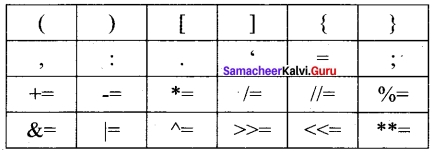Question 28.
Write note on Nested loop structure.
A loop placed within another loop is called as nested loop structure. One can place a while within another while; for within another for; for within while and while within for to construct such nested loops.
Following is an example to illustrate the use of for loop to print the following pattern
1
12
123
1234
12345

Question 29.
Write a program to display the sum of natural numbers upto n.
n = input (“Enter any number”)
sum = 0
for i in range (i, n+1):
sum = sum + i
print “sum = “, sum
Output:
Enter any number 5
sum = 15

Question 30
Differentiate list and dictionary.

 list dictionary 1. List is an ordered set of elements. A dictionary is a data structure that is used for matching one element (Key) with another (Value). 2. The index values can be used to access a particular element. In dictionary key represents index. Remember that, key may be a number of a string. 3. Lists are used to look up a value. A dictionary is used to take one value and look up another value.

Question 31.
Explain the commands which comes under TCL.
SQL command which come under Transfer Control Language are:

1. Commit Saves any transaction into the database permanently.
2. Roll back Restores the database to last commit state.
3. Save point Temporarily save a transaction so that you can rollback.

Question 32.
Differentiate Python from C++.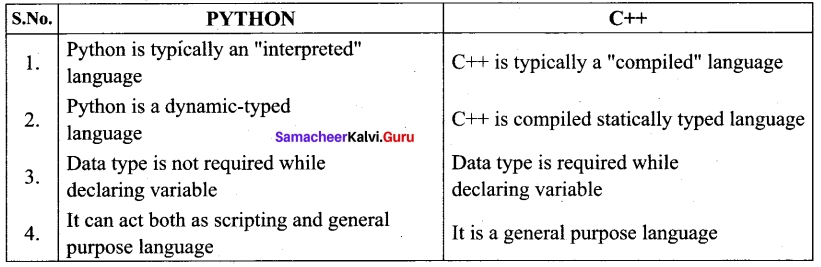Question 33.
Give the Pseudo code for Bubble sort algorithm.
Pseudo code

1. Start with the first element i.e., index = 0, compare the current element with the next element of the array.
2. If the current element is greater than the next element of the array, swap them.
3. If the current element is less than the next or right side of the element, move to the next element. Go to Step 1 and repeat until end of the index is reached.

PART – IV

Answer all the questions. [5 x 5 = 25]

Question 34 (a).
Explain the rules to be followed to format data in a CSV file.
Rules to be followed to format data in a CSV file
1. Each record (row of data) is to be located on a separate line, delimited by a line break by pressing enter key.
For example: ↵
xxx, yyy ↵
↵ denotes enter Key to be pressed

2. The last record in the file may or may not have an ending line break.
For example:
ppp, qqq ↵
yyy, xxx

3. There may be an optional header line appearing as the first line of the file with the same format as normal record lines. The header will contain names corresponding to the fields in the file and should contain the same number of fields as the records in the rest of the file.
For example: field_namel, field_name2, field_name3 ↵
aaa,bbb,ccc ↵
zzz, yyy, xxx CRLF (Carriage Return and Line Feed)

4. Within the header and each record, there may be one or more fields, separated by commas. Spaces are considered part of a field and should not be ignored. The last field in the record must not be followed by a comma. For example: Red , Blue

5. Each field may or may not be enclosed in double quotes. If fields are not enclosed with double quotes, then double quotes may not appear inside the fields.
For example: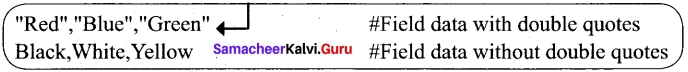6. Fields containing line breaks (CRLF), double quotes, and commas should be enclosed in double-quotes.
For example:
Red, ”, “, Blue CRLF # comma itself is a field value. So it is enclosed with double quotes Red, Blue , Green.

7. If double-quotes are used to enclose fields, then a double-quote appearing inside a field ‘ must be preceded with another double quote.
For example:
“Red, ” “Blue”, “Green”, # since double quotes is a field value it is enclosed with another double quotes,, White

[OR]

(b) Write a program to add a prefix text to all the lines in a string.
import textwrap
text =
‘”Strings are immutable. Slice is a
substring of a main string. Stride is a third argument in slicing operation'”
text_without_lndentation = textwrap.dedent(text)
wrapped = textwrap.fill(text_without_Indentation, width = 50)
print(textwrap.indent(wrapped, ‘*’)
print()
Output:

1. Strings are immutable. Slice is a
2. substring of a main string. Stride
3. is a third argument in slicing operation.

Question 35 (a).
Explain the purpose of range with an example.
The range( ) is a function used to generate a series of values in Python. Using range( ) function, you can create list with series of values. The range() function has three arguments.

Syntax of range () function:
range (start value, end value, step value)
where,
start value – beginning value of series. Zero is the default beginning value.
end value – upper limit of series. Python takes the ending value as upper limit – 1.
step value – It is an optional argument, which is used to generate different interval of values.
Example:
Generating whole numbers upto 10
for x in range (1, 11):
print(x)
Output
1
2
3
4
5
6
7
8
9
10[OR]

(b) Explain the various processing skills of SQL.
The various processing skills of SQL are :

1. Data Definition Language (DDL) : The SQL DDL provides commands for defining relation schemes (structure), deleting relations, creating indexes and modifying relation schemes.
2. data Manipulation Language (DML) : The SQL DML includes commands to insert, delete, and modify tuples in the database.
3. Embedded Data Manipulation Language : The embedded form of SQL is used in high level programming languages.
4. View Definition : The SQL also includes commands for defining views of tables.
5. Authorization : The SQL includes commands for access rights to relations and views of tables.
6. Integrity : The SQL provides forms for integrity checking using condition.
7. Transaction control : The SQL includes commands for file transactions and control over transaction processing.

Question 36 (a).
Explain various set operations.
As we leamt in mathematics, the python is also supports the set operations such as Union, Intersection, difference and Symmetric difference.

(i) Union: It includes all elements from two or more sets
In python, the operator | is used to union of two sets. The function union() is also used to join two sets in python.
Example:
Program to Join (Union) two sets using union operator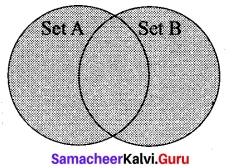set_A = {2, 4, 6, 8}
set_B = {‘A’, ‘B’, ‘C, ‘D’}
U_set = set_A| set_B
print(U_set)
Output:
{2, 4, 6, 8, ‘A’, ‘D’, ‘C, ’B’}

(ii) Intersection: It includes the common elements in two sets.
The operator & is used to intersect two sets in python. The function intersection ) is also used to intersect two sets in python.
Example: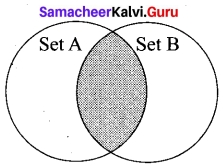Program to insect two sets using intersection operator
set_A = {‘A’, 2, 4, ‘D’}
set_B = {‘A’, ‘B’, ‘C’, ‘D’}
print(set_A & set_B)
Output:
{‘A’, ‘D’}

(iii) Difference: It includes all elements that are in first set (say set A) but not in the second set (say set B)
The minus (-) operator is used to difference set operation in python.
The function difference() is also used to difference operation.
Example: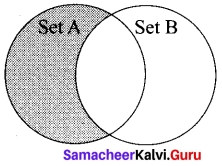Program to difference of two sets using minus operator
set_A = {’A’, 2, 4, ’D’}
set_B = {‘A’, ’B’, ‘C’, ’D’}
print(set_A – set_B)
Output:
{2, 4}

(iv) Symmetric difference: It includes all the elements that are in two sets (say sets A and B) but not the one that are common to two sets.
The caret (^) operator is used to symmetric difference set operation in python. The function symmetric_difference() is also used to do the same operation.
Example: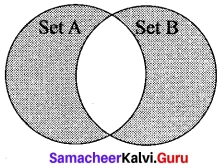Program to symmetric difference of two sets using caret operator
set_A = {‘A’, 2, 4, ‘D’}
set_B = {‘A’, ‘B’, ’C’, ‘D’}
print(set_A ^ set_B)
Output:
{2, 4, ’B’, ‘C’}[OR|

(b) Write a program that accept a string from the user and display the same after removing vowels from it.
def rem_vowels(s):
temp_str = ”
for i in s:
if i in “aAeEiIoOuU”:
pass
else:
temp_str+ = i
print (“The string without vowels: “, temp str)
strl= input (“Enter a String: “)
rem_vowels (strl)
Output:
Enter a String: Mathematical foundations of Computer Science The string without vowels: Mthmtcl fndtns f Cmptr Scnc

Question 37 (a).
Write a program to display all records using fetchall().
The fetchall() method is used to fetch all rows from the database table
import sqlite3
cursor = connection.cursor()
cursor.execute(“SELECT * FROM student”)
print(“fetchall:”)
result = cursor.fetchall()
for r in result:
print(r)
Output:
fetchall:
(1, ‘Akshay’, ‘B’, ‘M’, 87.8, ’2001-12-12′)
(2, ‘Aravind’, ’A’, ‘M’, 92.5, ‘2000-08-17’)
(4, ‘SAJINT, ‘A’, ‘F’, 95.6, ‘2002-11-01’)
(5, ‘VARUN’,’B’, ‘M’, 80.6,’2001-03-14′)
(6, ‘PRIYA’, ‘A’, ‘F’, 98.6, ‘2002-01-01’)
(7, ‘TARUN’, ‘D’, ‘M’, 62.3, ‘1999-02-01’)

[OR]

(b) Write a C++ program using user defined function to find cube of a number.
#include <iostream>
using namespace std;
// Function declaration
int cube(int num);
int main()
{
int num;
int c;
cout <<“Enter any number: “<<endl;
cin>>num;
c = cube(num);
cout<<“Cube of” <<num<<” is “<<c;
return 0;
}
//Function to find cube of any number
int cube(int num)
{
return (num * num * num);
}
// Save this file as cubefile.cpp
#Now select File→New in Notepad and type the Python program
# Save the File as fun.py
# Program that compiles and executes a .cpp file
# Python fun.py -i c:\pyprg\cube_file.cpp
import sys, os, getopt
def main (argv):
cppfile = ”
exefile = ”
opts, args = getopt.getopt(argv, “i:”,[‘ifile=’])
for o, a in opts:
if o in (“-i”, “–ifile”):
cpp_file = a + ‘.cpp’
exe_file = a + ‘.exe’
run (cpp_file, exe_file)
def run (cpp_file, exe_file):
print(“Compiling” + cpp file)
os.system (‘g++ ‘ + cpp_file +’ -o ‘ + exe_file)
print (“Running ” + exe_file)
print(“———-“)
print
os.system(exe_file)
print
if_name_ == ‘_main_’:
main(sys.argv[l:])
Output of the above program
Compiling c:\pyprg\cube_file.cpp
Running c:\pyprg\cube_file.exe
————–
Enter any number:
5
Cube of 5 is 125Question 38 (a).
Explain reverse Indexing in list using python program.
Python enables reverse or negative indexing for the list elements. Thus, python lists index in opposite order. The python sets -1 as the index value for the last element in list and -2 for the preceding element and so on. This is called as Reverse Indexing.
Example:
Marks = [10, 23, 41, 75]
i = -1
while i > = -4:
print (Marks[i])
i = i + -l
Output
75
41
23
10

[OR]

(b) Explain sort function in list with examples.
Sorts the element in list
Both arguments are optional
1. If reverse is set as True, list sorting is in descending order.
2. Ascending is default.
3. Key=myFunc; “myFunc” – the name of the user defined function that specifies the sorting criteria.
MyList = [‘Thilothamma’, ‘Tharani’, ‘Anitha’, ‘SaiSree’, ‘Lavanya’]
MyList.sort( )
print (MyList)
MyList.sort(reverse = True)
print (MyList)
Output:.
[‘Anitha’, ‘Lavanya’, ‘SaiSree’, ‘Tharani’, ‘Thilothamma’]
[‘Thilothamma’, ‘Tharani’, ‘SaiSree’, ‘Lavanya’, ‘Anitha’]